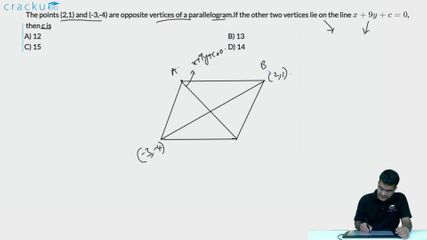Question 65

# The points (2,1) and (-3,-4) are opposite vertices of a parallelogram.If the other two vertices lie on the line $$x+9y+c=0$$, then c is

Solution

The midpoints of two diagonals of a parallelogram are the same

Hence the midpoint of (2,1) and (-3,-4) lie on $$x+9y+c=0$$

midpoint of (2,1) and (-3,-4) = ($$\frac{2-3}{2},\frac{1-4}{2}$$) = (-1/2 , -3/2)

Keeping this cordinates in the above line equation, we get c = 14

### View Video Solution